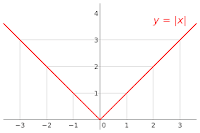## Friday, September 02, 2016

### Friday Science: Real Number Calculus

This is my post on the sixth chapter.

1. I'm sure there is a method to Penrose's madness. He's giving the building blocks and later he'll build. This chapter was about real number calculus. He gave a brief intro to differentiation and integration.

Basically, differentiation is the process of finding a function that describes the slope of another function at every point. For example, the function u=2x describes the slope of the function y=x2 at every point. He gives the most basic formulas for figuring these slope formulas out without explanation.

2. Integration goes the other way. If you are told that u=2x is the derivative of some other function, integration figures out that the function in view is y = x2 + some constant. In the case above that constant was 0, but 2x could be the derivative of an infinite number of other instances, like y=x2 + 523 . Don't worry about it if you don't know calculus. Not important. The integral of a function turns out basically to be the area under it.absolute value function, Wikipedia
3. He spends a lot of time talking about functions that Leonhard Euler would have liked (pronounced OIL-er, 1707-83). Interestingly, he doesn't really give the definition I learned. He looks at functions that are continuous, functions that are differentiable. He tells us Euler probably wouldn't have liked the absolute value function, step functions, or piecewise functions.

But Euler would have liked the hyperbolic function y=1/x, even though it doesn't have a value for x=0.

4. Apparently Euler liked functions that could be expressed as power series.from mathwords.com

Again, I'm sure there's a reason Penrose is covering this particular territory. I look forward to finding out what it is. :-)

5. The theta function or Heaviside step function is a function that is 0 for any number less than 0 and 1 for any number greater than zero. He introduces the derivative of the theta function as the Dirac delta function. It looks like it will be significant for quantum mechanics later. The Dirac delta function is zero everywhere except for zero. At zero, the function is like an infinite line going up. The strange thing is that the "area" of that infinite spike is one.

I'm just going to leave it at that.